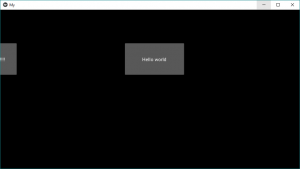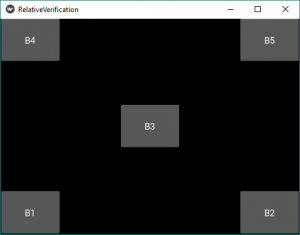# Python | Relative Layout in Kivy

Kivy is a platform-independent GUI tool in Python. As it can be run on Android, IOS, Linux and Windows, etc. It is basically used to develop the Android application, but it does not mean that it can not be used on Desktop applications.

### Relative Layout:

• Relative layout is just similar to the FloatLayout the difference is that its child widget are positioned relative to the layout.
• This layout operates in the same way as FloatLayout does, but the positioning properties (x, y, center_x, right, y, center_y, and top) are relative to the Layout size and not the window size.
• In reality regardless of absolute and relative positioning, the widgets are moved when the position of the layout changes.
• When the widget with position=(0, 0) is added to RelativeLayout, Now if the position of RelativeLayout is changed the child widget will also move. The Child widget coordinates remains same i.e (0, 0) as they are always relative to the parent layout.
• The available pos_hint keys (x, center_x, right, y, center_y, and top) are useful for aligning to edges or centering.
For example:
pos_hint: {‘center_x’:.5, ‘center_y’:.5} would align a Widget in the middle, no matter what the size of the window is.

The first thing we need to do to use a RelativeLayout is to import it.

`from kivy.uix.relativelayout import RelativeLayout`

Note:
This layout allows you to set relative coordinates for children. If you want absolute positioning, use the FloatLayout. In RelativeLayout each child widget size and position has to be given. This also does the dynamic placement.

```We can do relative positioning by:
pos_hint: provide hint of position
We can define upto 8 keys i.e. it takes arguments in form of dictionary.
pos_hint = {“x”:1, “y”:1, “left”:1, “right”:1, "center_x":1, "center_y":1,
“top”:1, “bottom”:1("top":0)}```

Note:
`Floatlayout `and `RelativeLayout `both support absolute and relative positioning depending upon whether pos_hint or pos is used. But If you want absolute positioning, use the FloatLayout.

```Basic Approach to create Relative Layout:

1) import kivy
2) import kivyApp
3) import button
4) import Relativelayout
5) Set minimum version(optional)
6) create App class:
- define build() function
7) return Layout/widget/Class(according to requirement)
8) Run an instance of the class```

Implementation of approach using pos :
It simply assigns the position to the button. As `Relativelayout `does not depends on the window size it gets fixed to that position now if you do the window size small it may disappear rather than adjusting itself.

 `# Sample Python application demonstrating the  ` `# working of RelativeLayout in Kivy  ` ` `  `# import modules ` `import` `kivy  ` ` `  `# base Class of your App inherits from the App class.  ` `# app:always refers to the instance of your application  ` `from` `kivy.app ``import` `App  ` ` `  `# creates the button in kivy  ` `# if not imported shows the error  ` `from` `kivy.uix.button ``import` `Button  ` ` `  `# This layout allows you to set relative coordinates for children. ` `from` `kivy.uix.relativelayout ``import` `RelativeLayout ` ` `  `# To change the kivy default settings  ` `# we use this module config  ` `from` `kivy.config ``import` `Config  ` `     `  `# 0 being off 1 being on as in true / false  ` `# you can use 0 or 1 && True or False  ` `Config.``set``(``'graphics'``, ``'resizable'``, ``True``)  ` ` `  `# creating the App class  ` `class` `MyApp(App):  ` ` `  `    ``def` `build(``self``):  ` ` `  `        ``# creating Relativelayout  ` `        ``Rl ``=` `RelativeLayout()  ` ` `  `        ``# creating button  ` `        ``# a button 30 % of the width and 20 %  ` `        ``# of the height of the layout and  ` `        ``# positioned at (x, y), you can do ` `        ``# The position does not depend on window size ` `        ``# it just positioned at the given places:  ` `        ``btn ``=` `Button(text ``=``'Hello world'``,  ` `                 ``size_hint ``=``(.``2``, .``2``),  ` `                 ``pos ``=``(``396.0``, ``298.0``)) ` `        ``btn1 ``=` `Button(text ``=``'Hello world !!!!!!!!!'``,  ` `                 ``size_hint ``=``(.``2``, .``2``),  ` `                 ``pos ``=``(``-``137.33``, ``298.0``)) ` ` `  `        ``# adding widget i.e button  ` `        ``Rl.add_widget(btn) ` `        ``Rl.add_widget(btn1) ` `                 `  `        ``# return the layout  ` `        ``return` `Rl  ` ` `  `# run the App  ` `if` `__name__ ``=``=` `"__main__"``:  ` `    ``MyApp().run()  `

Output:Now if you want that the button adjusts itself according to window pos_hint is used.

Implementation Approach by using pos_hint

 `# Sample Python application demonstrating the  ` `# working of RelativeLayout in Kivy  ` ` `  `# import modules ` `import` `kivy  ` ` `  `# base Class of your App inherits from the App class.  ` `# app:always refers to the instance of your application  ` `from` `kivy.app ``import` `App  ` ` `  `# creates the button in kivy  ` `# if not imported shows the error  ` `from` `kivy.uix.button ``import` `Button  ` ` `  `# This layout allows you to set relative coordinates for children. ` `from` `kivy.uix.relativelayout ``import` `RelativeLayout ` ` `  `# To change the kivy default settings  ` `# we use this module config  ` `from` `kivy.config ``import` `Config  ` `     `  `# 0 being off 1 being on as in true / false  ` `# you can use 0 or 1 && True or False  ` `Config.``set``(``'graphics'``, ``'resizable'``, ``True``)  ` ` `  `# creating the App class  ` `class` `Relative_Layout(App): ` `     `  `    ``def` `build(``self``): ` ` `  `        ``# creating Relativelayout  ` `        ``rl ``=` `RelativeLayout() ` `       `  `        ``# creating button ` `        ``# size of button is 20 % by hight and width of layout ` `        ``# position is bottom left i.e x = 0, y = 0 ` `        ``b1 ``=` `Button(size_hint ``=``(.``2``, .``2``), ` `                    ``pos_hint ``=``{``'x'``:``0``, ``'y'``:``0``}, ` `                    ``text ``=``"B1"``) ` `         `  `        ``# position is bottom right i.e right = 1, y = 0 ` `        ``b2 ``=` `Button(size_hint ``=``(.``2``, .``2``), ` `                    ``pos_hint ``=``{``'right'``:``1``, ``'y'``:``0``}, ` `                    ``text ``=``"B2"``) ` ` `  `        ``b3 ``=` `Button(size_hint ``=``(.``2``, .``2``), ` `                    ``pos_hint ``=``{``'center_x'``:.``5``, ``'center_y'``:.``5``}, ` `                    ``text ``=``"B3"``) ` ` `  `        ``b4 ``=` `Button(size_hint ``=``(.``2``, .``2``), ` `                    ``pos_hint ``=``{``'x'``:``0``, ``'top'``:``1``}, ` `                    ``text ``=``"B4"``) ` ` `  `        ``b5 ``=` `Button(size_hint ``=``(.``2``, .``2``), ` `                    ``pos_hint ``=``{``'right'``:``1``, ``'top'``:``1``}, ` `                    ``text ``=``"B5"``) ` `         `  `         `  ` `  `        ``# adding button to widget ` `        ``rl.add_widget(b1) ` `        ``rl.add_widget(b2) ` `        ``rl.add_widget(b3) ` `        ``rl.add_widget(b4) ` `        ``rl.add_widget(b5) ` `     `  `         `  `        ``# returning widget ` `        ``return` `rl ` `# run the App  ` `if` `__name__  ``=``=` `"__main__"``: ` `    ``Relative_Layout().run() `

Output:My Personal Notes arrow_drop_upCheck out this Author's contributed articles.

If you like GeeksforGeeks and would like to contribute, you can also write an article using contribute.geeksforgeeks.org or mail your article to contribute@geeksforgeeks.org. See your article appearing on the GeeksforGeeks main page and help other Geeks.

Please Improve this article if you find anything incorrect by clicking on the "Improve Article" button below.

Article Tags :

Be the First to upvote.

Please write to us at contribute@geeksforgeeks.org to report any issue with the above content.